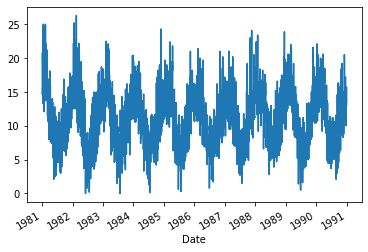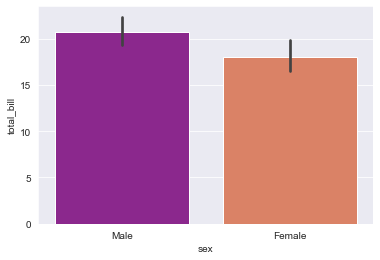## Write programs to demonstrate plotting Categorical and Time-Series Data.

A series of data points collected over the course of a time period, and that are time-indexed is known as Time Series data. These observations are recorded at successive equally spaced points in time. For Example, the ECG Signal, EEG Signal, Stock Market, Weather Data, etc., all are time-indexed and recorded over a period of time. Analyzing these data, and predicting future observations has a wider scope of research.

### Plotting Time series Data:

Example: Minimum Daily Temperatures Dataset

This dataset describes the minimum daily temperatures over 10 years (1981-1990) in the city Melbourne, Australia.

The units are in degrees Celsius and there are 3,650 observations. The source of the data is credited as the Australian Bureau of Meteorology.

Download the dataset and place it in the current working directory with the filename “daily-min-temperatures.csv“.

Below is an example of loading the dataset as a Panda Series.

from matplotlib import pyplot

Output:

```Date
1981-01-01    20.7
1981-01-02    17.9
1981-01-03    18.8
1981-01-04    14.6
1981-01-05    15.8
Name: Temp, dtype: float64```

plotting line chart for above dataset

from matplotlib import pyplot
series.plot()
pyplot.show()

Output:-### Plotting Categorical Data

What is Categorical Data ?

Categorical variables represent types of data which may be divided into groups. Examples of categorical variables are race, sex, age group, and educational level. While the latter two variables may also be considered in a numerical manner by using exact values for age and highest grade completed, it is often more informative to categorize such variables into a relatively small number of groups.

Analysis of categorical data generally involves the use of data tables. A two-way table presents categorical data by counting the number of observations that fall into each group for two variables, one divided into rows and the other divided into columns. For example, suppose a survey was conducted of a group of 20 individuals, who were asked to identify their hair and eye color. A two-way table presenting the results might appear as follows:

Here we are plotting for "tips" dataset

# import the seaborn library
import seaborn as sns

# import done to avoid warnings
from warnings import filterwarnings

# first five entries if the dataset

Output:-

Step-2: plotting above data set

# set the background style of the plot
sns.set_style('darkgrid')

# plot the graph using the default estimator mean
sns.barplot(x ='sex', y ='total_bill', data = df, palette ='plasma')

Output:-More

More

More

More

More

More
Top